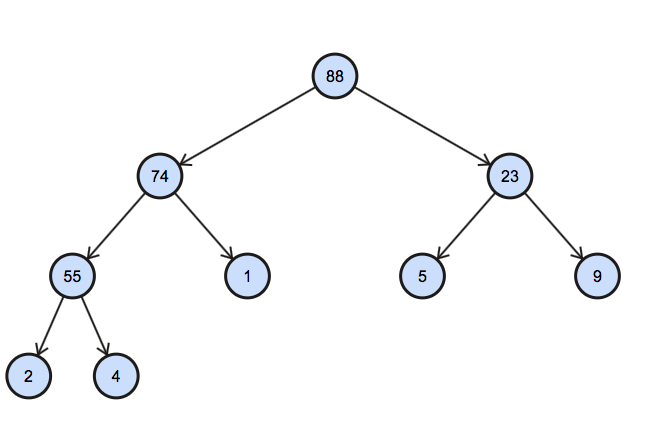# Random Wits

Life is too short for a diary

$latest projects ├── encrypted-files ├── binary-semaphore ├── AES └── DES+ view all  ## Random $ random stuff     ├── my bookshelf     ├── resources     └── quotes     └── about me

+ say Hello

Sun 20 Mar 2016

### Find kth largest element in an array

The other day I stumble upon the question to find the kth largest element in the array. At first glance, I thought the solution was trivial. But later I thought that there are multiple ways to achieve efficient solution

Heap is a useful data structure when root of the tree is important. Extracting an element from the heap is in logarithmic time. This could be used to find kth element from the array since finding maximum or minimum in heap is constant time.

# Problem Statement

Suppose, we have an array of arbitrary integer values. We need to find the kth largest element in the array. A sample of the input file is given below

3
9, 4, 5, 2, 1, 23, 55, 88, 74


Here, the first line is the value of n and next line contains the array

# Desired Output

The output should print the 3rd element in the array i.e.

55


# Step 1 aka Read the input file

Before we begin, we need to perpare our tools and gather required inputs. First we need to read the input file and get the value of k and the array. Also I’ll be using ruby to write code as it is closer to psuedo code. So fire up your text editor and paste the code below

# Step 2 aka the easy way

One of the first solution that pops in my mind to find the nth largest element is

1. Sort the array in descending order
2. Get the element at the kth index

The time complexity for this solution is $O(n\log{}n)$ . However we can still find another solution in $O(n)$ time.

# More Efficiently?

We can visualize our array as tree with each value as a node of tree. However to build our array as heap , we need to satisfy certain properties

1. Each node of the heap has value
2. Value of each node $\ge$ values of each of its children (Max-heap)

In a nutshell our array should look like this

88,74,23,55,1,5,9,2,4## Building the heap

Building heap takes O(n) time. First visit to every non-leaf node and checks if the value at the node satisfy heap property.

Max_down() is the most important function as it heapifies (or maintain the heap property).

## Extract Max

Our last step involves extracting the kth max element.

1. Extract the fist element which is the root element $O(1)$
2. Call heapify to maintain heap property $O(\log{}(n)$
3. Call extract k times extract max $O(k \log{}(n))$

# Conclusion

Time complexity analysis of two methods discussed above

 Using Worst Case Description Sort O(n log(n)) + O(1) Sorting the array + accessing the element Heap O(n) + O(k log(n)) Building heap + extracting maximum element